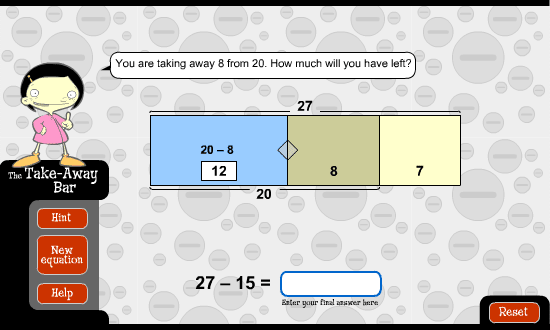﻿ The take-away bar: go figure: round to the nearest 10Round to the nearest 10

The idea behind this strategy is to use subtraction to round a number down to its nearest 10. Then you take away the remaining amount to get the final answer.

First, work out how to break up 8 in order to take 54 to its nearest 10. In this case, it's 4 and 4. Next, take away the 4 from 54 to make 50. Then, take away the remaining 4 to give the answer of 46.Now let's use this strategy to work out 27 minus 15. This time, break 15 into 7 and 8. Next, take away 7 from 27 to make 20. Then, take away the remaining 8 to give the answer 12.Let's try this strategy to work out 53 minus 8. In order to take 53 to its nearest ten, first break up 8 into 3 and 5. Next, take away 3 from 53 to make 50. Then, take away 5 from that.

Did you get 45?